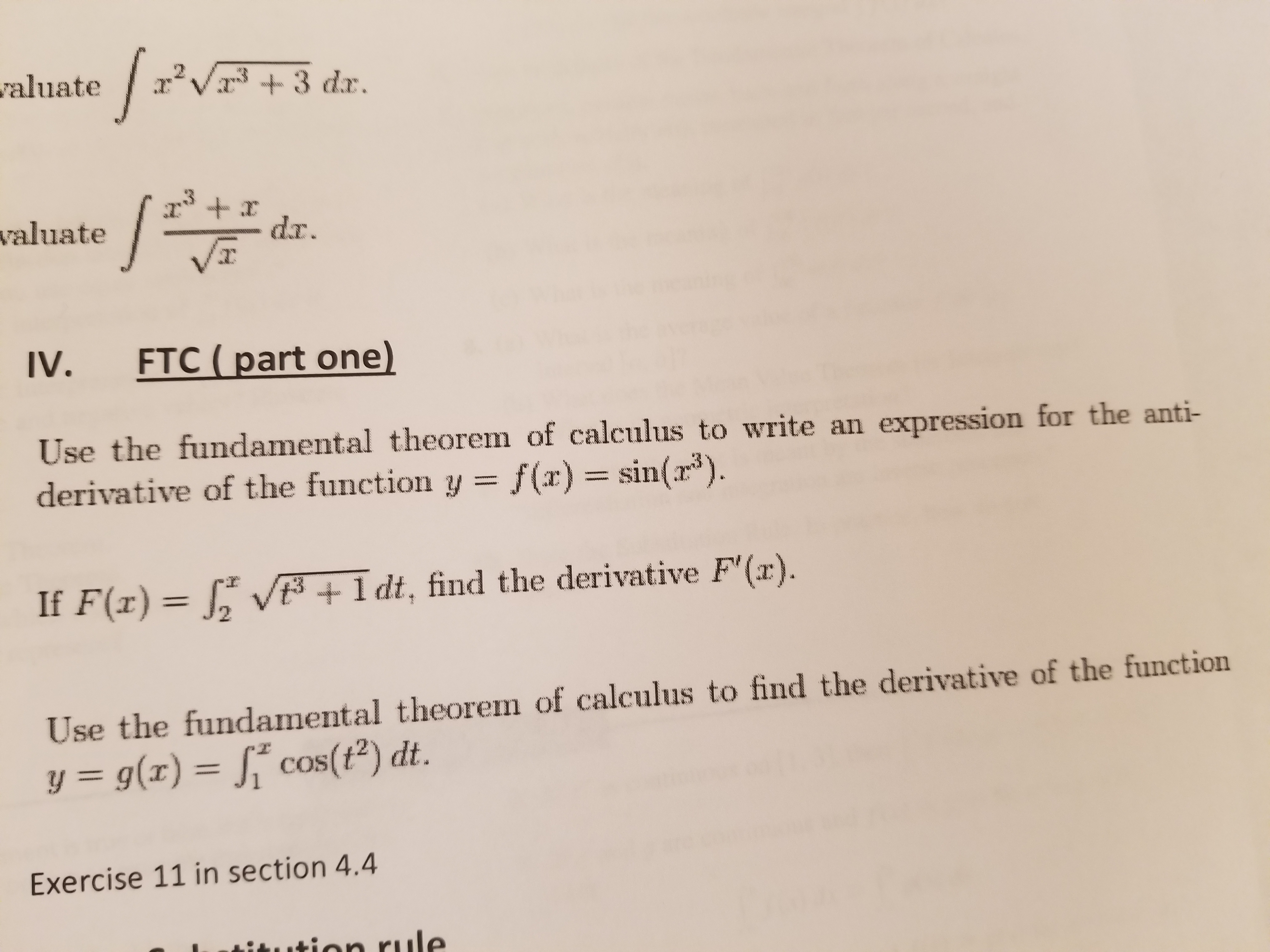# abuate3 dar.valuateVTIV.FTC (part one)Use the fundamental theorem of calculus to write an expression for the anti-derivative of the function y = f(x) = sin(13).If F(x)12 V t+ldt, find the derivative F'(x).Use the fundamental theorem of calculus to find the derivative of the functiony = g(x)-j" cos(t2) dt.Exercise 11 in section 4.4

Question
1 views

I am studying for a quiz and do not understand a few of the concepts that will be covered on it. Can you help me with the problem under "IV.  FTC (part one)" found in the attached document?  Thank you.help_outlineImage Transcriptioncloseabuate3 dar. valuate VT IV. FTC (part one) Use the fundamental theorem of calculus to write an expression for the anti- derivative of the function y = f(x) = sin(13). If F(x) 12 V t +ldt, find the derivative F'(x). Use the fundamental theorem of calculus to find the derivative of the function y = g(x)-j" cos(t2) dt. Exercise 11 in section 4.4 fullscreen
check_circle

Step 1

The fundamental theorem of calculus states that for a continuous real-valued function, f on a closed interval [a, b], let the function F be defined, for all x in [a, b] as

Step 2

Here, the function is given as y=sin(x3)  which is continuous on any closed interval, hence consider a closed interval [a,b]. Use the fundamental theorem of calculus and obtain the antiderivative F as follows.

Step 3

The antiderivative F which is  uniformly c...

### Want to see the full answer?

See Solution

#### Want to see this answer and more?

Solutions are written by subject experts who are available 24/7. Questions are typically answered within 1 hour.*

See Solution
*Response times may vary by subject and question.
Tagged in

### Continuity